onlyloveyd 503 5

## 霍夫直线检测

### 点和线的对偶性

• 图像空间中的点，对应霍夫空间中的直线

• 图像空间中的直线，对应霍夫空间中的点

• 共点的直线，在霍夫空间中对应的点在一条直线上

• 共线的点，在霍夫空间中对应的直线交于一点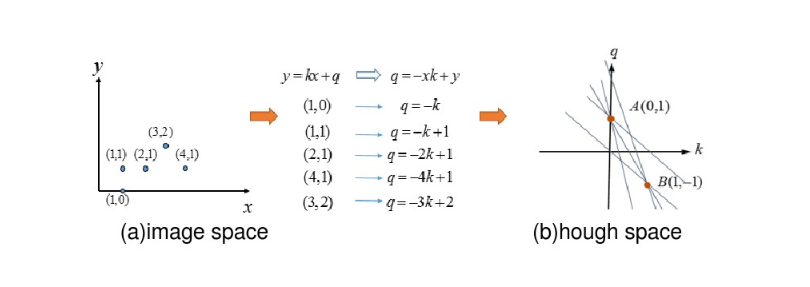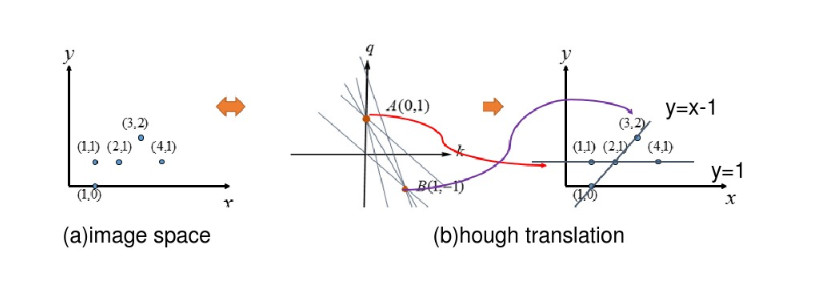### 极坐标参数方程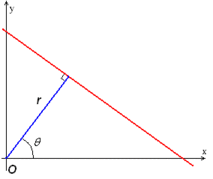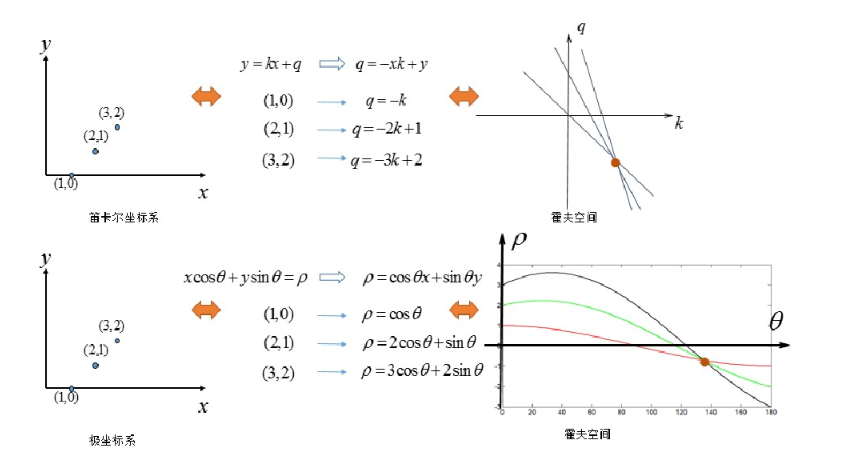• 将参数空间的坐标轴离散化，例如theta=0,10,20……， r=0.1,0.2,0.3……
• 将图像中每个非0像素通过映射关系求取在参数空间通过的方格。
• 统计参数空间内每个方格出现的次数，选取次数大于某一阈值的方格作为表示直线的方格。
• 将参数空间中表示直线的方格的参数作为图像中直线的参数。

## API

public static void HoughLines(Mat image, Mat lines, double rho, double theta, int threshold, double srn, double stn, double min_theta)
• 参数一：image，待检测直线的原图像，必须是CV_8U的单通道图像.

• 参数二：lines，霍夫变换检测到的直线输出量，每一条直线都由两个或者三个参数表示。第一个表示直线距离坐标原点的距离 ，第二个表示坐标原点到直线的垂线与x轴的夹角，若有第三个，则表示累加器的数值。 $$((\rho, \theta)) or ((\rho, \theta, \textrm{votes}))$$

• 参数三：rho，距离分辨率，以像素为单位，距离离散化时的单位长度

• 参数四：theta，角度分辨率，以弧度为单位，夹角离散化时的单位角度。

• 参数五：threshold，累加器的阈值，即参数空间中离散化后每个方格被通过的累计次数大于该阈值时将被识别为直线，否则不被识别为直线。

• 参数六：srn，对于多尺度霍夫变换算法中，该参数表示距离分辨率的除数，粗略的累加器距离分辨率是第三个参数rho，精确的累加器分辨率是rho/srn。这个参数必须是非负数，默认参数为0。

• 参数七：stn，对于多尺度霍夫变换算法中，该参数表示角度分辨率的除数，粗略的累加器距离分辨率是第四个参数rho，精确的累加器分辨率是rho/stn。这个参数必须是非负数，默认参数为0。当这个参数与第六个参数srn同时为0时，此函数表示的是标准霍夫变换。

• 参数八：min_theta，检测直线的最小角度，默认参数为0。

• 参数九：max_theta，检测直线的最大角度，默认参数为CV_PI，是OpenCV 4中的默认数值具体为3.1415926535897932384626433832795。

public static void HoughLinesP(Mat image, Mat lines, double rho, double theta, int threshold, double minLineLength, double maxLineGap) 
• 参数一：image，待检测直线的原图像，必须是CV_8U的单通道图像.

• 参数二：lines，输出线段。每条线由4元素表示。如下，分别代表每个线段的两个端点 $$(x_1, y_1, x_2, y_2)$$

• 参数三：rho，距离分辨率，以像素为单位，距离离散化时的单位长度

• 参数四：theta，角度分辨率，以弧度为单位，夹角离散化时的单位角度。

• 参数五：threshold，累加器的阈值，即参数空间中离散化后每个方格被通过的累计次数大于该阈值时将被识别为直线，否则不被识别为直线。该累积数越大，则得到的直线可能就越长。

• 参数六：minLineLength，表示可以检测的最小线段长度，根据实际需要进行设置。

• 参数七：maxLineGap，表示线段之间的最大间隔像素，假设5表示小于5个像素的两个相邻线段可以连接起来。

## 操作

package cn.onlyloveyd.demo.ui

import android.os.Bundle
import androidx.appcompat.app.AppCompatActivity
import androidx.databinding.DataBindingUtil
import cn.onlyloveyd.demo.R
import cn.onlyloveyd.demo.databinding.ActivityHoughLineBinding
import cn.onlyloveyd.demo.ext.showMat
import org.opencv.android.Utils
import org.opencv.core.Mat
import org.opencv.core.Point
import org.opencv.core.Scalar
import org.opencv.imgproc.Imgproc
import kotlin.math.cos
import kotlin.math.roundToInt
import kotlin.math.sin

/**
* 霍夫直线检测
* author: yidong
* 2020/7/18
*/
class HoughLineDetectActivity : AppCompatActivity() {

private lateinit var mBinding: ActivityHoughLineBinding
private lateinit var mGray: Mat
private lateinit var mEdge: Mat
override fun onCreate(savedInstanceState: Bundle?) {
super.onCreate(savedInstanceState)
mBinding = DataBindingUtil.setContentView(this, R.layout.activity_hough_line)
mBinding.presenter = this
mGray = Mat()
mEdge = Mat()
Imgproc.cvtColor(bgr, mGray, Imgproc.COLOR_BGR2GRAY)
mBinding.ivLena.showMat(mGray)
Imgproc.Canny(mGray, mEdge, 80.0, 150.0, 3, false)
}

override fun onDestroy() {
mGray.release()
mEdge.release()
super.onDestroy()
}

fun doHoughLineDetect() {
title = "HoughLine"
val lines = Mat()
Imgproc.HoughLines(mEdge, lines, 1.0, Math.PI / 180.0, 150)
val out = Mat.zeros(mGray.size(), mGray.type())
val data = FloatArray(2)
for (i in 0 until lines.rows()) {
lines.get(i, 0, data)
val rho = data // 直线距离坐标原点的距离
val theta = data // 直线过坐标原点垂线与x轴夹角
val a = cos(theta.toDouble())  //夹角的余弦值
val b = sin(theta.toDouble())  //夹角的正弦值
val x0 = a * rho  //直线与过坐标原点的垂线的交点
val y0 = b * rho
val pt1 = Point()
val pt2 = Point()
pt1.x = (x0 + 1000 * (-b)).roundToInt().toDouble()
pt1.y = (y0 + 1000 * (a)).roundToInt().toDouble()
pt2.x = (x0 - 1000 * (-b)).roundToInt().toDouble()
pt2.y = (y0 - 1000 * (a)).roundToInt().toDouble()
Imgproc.line(out, pt1, pt2, Scalar(255.0, 255.0, 255.0), 2, Imgproc.LINE_AA, 0)
}
mBinding.ivResult.showMat(out)
out.release()
lines.release()
}

fun doHoughLinePDetect() {
title = "HoughLineP"
val lines = Mat()
Imgproc.HoughLinesP(mEdge, lines, 1.0, Math.PI / 180.0, 100, 50.0, 10.0)
val out = Mat.zeros(mGray.size(), mGray.type())
for (i in 0 until lines.rows()) {
val data = IntArray(4)
lines.get(i, 0, data)
val pt1 = Point(data.toDouble(), data.toDouble())
val pt2 = Point(data.toDouble(), data.toDouble())
Imgproc.line(out, pt1, pt2, Scalar(255.0, 255.0, 255.0), 2, Imgproc.LINE_AA, 0)
}
mBinding.ivResult.showMat(out)
out.release()
lines.release()
}
}

## 效果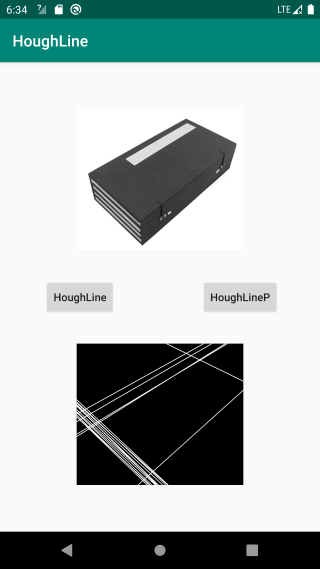## 源码

https://github.com/onlyloveyd/LearningAndroidOpenCV